Example Questions

Example Question #14 : How To Find The Equation Of A Perpendicular Line

In the xy-plane, the equation of the line n is –x+8y=17. If the line m is perpendicular to line n, what is a possible equation of line m?

x= -8y + (17/8)

y= 8x-17

y= -1/8x + 5

y= -8x + 5

y= -8x + 5

Explanation:

We start by add x to the other side of the equation to get the y by itself, giving us 8y =17 + x. We then divide both sides by 8, giving us y= 17/8 + 1/8x. Since we are looking for the equation of a perpendicular line, we know the slope (the coefficient in front of x) will be the opposite reciprocal of the slope of our line, giving us y= -8x + 5 as the answer.

Example Question #1 : How To Find The Equation Of A Perpendicular Line

What line is perpendicular to x + 3y = 6 and travels through point (1,5)?

y = 2x + 1

y = 2/3x + 6

y = 3x + 2

y = 6x – 3

y = –1/3x – 4

y = 3x + 2

Explanation:

Convert the equation to slope intercept form to get y = –1/3x + 2.  The old slope is –1/3 and the new slope is 3.  Perpendicular slopes must be opposite reciprocals of each other:  m1 * m2 = –1

With the new slope, use the slope intercept form and the point to calculate the intercept: y = mx + b or 5 = 3(1) + b, so b = 2

So y = 3x + 2

Example Question #1 : How To Find The Equation Of A Perpendicular Line

What line is perpendicular to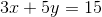and passes through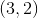?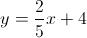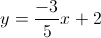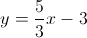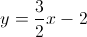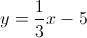Explanation:

Convert the given equation to slope-intercept form.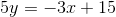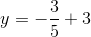The slope of this line is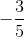. The slope of the line perpendicular to this one will have a slope equal to the negative reciprocal.

The perpendicular slope is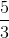.

Plug the new slope and the given point into the slope-intercept form to find the y-intercept.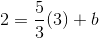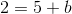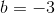So the equation of the perpendicular line is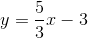.

Example Question #4 : How To Find The Equation Of A Perpendicular Line

Line m passes through the points (1, 4) and (5, 2). If line p is perpendicular to m, then which of the following could represent the equation for p?

x  y = 3

2x  y = 3

4x  3y = 4

2x + y = 3

3x + 2y = 4

2x  y = 3

Explanation:

The slope of m is equal to   y2-y1/x2-x1  =  2-4/5-1 -1/2

Since line p is perpendicular to line m, this means that the products of the slopes of p and m must be 1:

(slope of p) * (-1/2) = -1

Slope of p = 2

So we must choose the equation that has a slope of 2. If we rewrite the equations in point-slope form (y = mx + b), we see that the equation 2x  y = 3 could be written as y = 2x – 3. This means that the slope of the line 2x – y =3 would be 2, so it could be the equation of line p. The answer is 2x – y = 3.

Example Question #5 : How To Find The Equation Of A Perpendicular Line

What is the equation for the line that is perpendicular to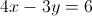through point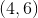?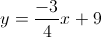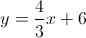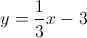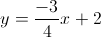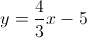Explanation:

Perpendicular slopes are opposite reciprocals.

The given slope is found by converting the equation to the slope-intercept form.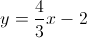The slope of the given line is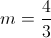and the perpendicular slope is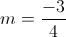.

We can use the given point and the new slope to find the perpendicular equation. Plug in the slope and the given coordinates to solve for the y-intercept.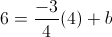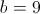Using this y-intercept in slope-intercept form, we get out final equation:.

Example Question #6 : How To Find The Equation Of A Perpendicular Line

Which line below is perpendicular to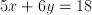?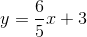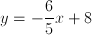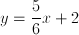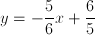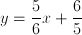Explanation:

The definition of a perpendicular line is one that has a negative, reciprocal slope to another.

For this particular problem, we must first manipulate our initial equation into a more easily recognizable and useful form: slope-intercept form or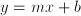.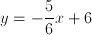According to ourformula, our slope for the original line is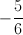. We are looking for an answer that has a perpendicular slope, or an opposite reciprocal. The opposite reciprocal ofis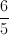. Flip the original and multiply it by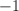Our answer will have a slope of. Search the answer choices forin the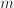position of theequation.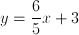is our answer.

(As an aside, the negative reciprocal of 4 is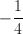. Place the whole number over one and then flip/negate. This does not apply to the above problem, but should be understood to tackle certain permutations of this problem type where the original slope is an integer.)

Example Question #7 : How To Find The Equation Of A Perpendicular Line

If a line has an equation of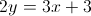, what is the slope of a line that is perpendicular to the line?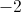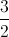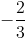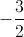Explanation:

Putting the first equation in slope-intercept form yields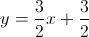.

A perpendicular line has a slope that is the negative inverse. In this case,.

Example Question #21 : How To Find The Equation Of A Perpendicular Line

Which of the following is NOT perpendicular to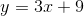?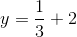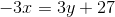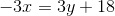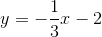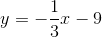Explanation:

Parallel lines have slopes that are opposite reciprocals. Any line perpendicular tomust have a slope of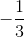.  The only line that doesn't have this slope is, which has a slope of.

Example Question #22 : How To Find The Equation Of A Perpendicular Line

A vertical line is drawn to connect the x-axis and point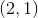.  What must be the equation of the perpendicular line that connects to this point?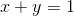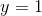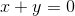Explanation:

The given key word is vertical.  If a perpendicular line that intersects the vertical line, the slope must be zero, and the line would be a horizontal line.  The horizontal line will intersect the point at, which means that the y-value will never change.

The answer is:Example Question #23 : How To Find The Equation Of A Perpendicular Line

Which of the following is perpendicular to the line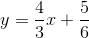?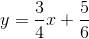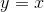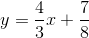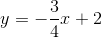Explanation:

First, we want to identify the slope of our given line. In slope-intercept form, a line's slopeis the coefficient ofThe slope of our given line isNow, we can start to find the perpendicular line. Remember that in order for two lines to be perpendicular, their slopes must be negative reciprocals of each other.

In this case, we want to find a line whose slope is the negative reciprocal of. So, we want a line whose slope is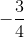With this in mind, the only answer choice with a slope ofisTherefore, the perpendicular line is